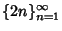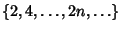## Sequence

A sequence is an ordered set of mathematical objects which is denoted using braces. For example, the symboldenotes the infinite sequence of Even Numbers.

See also 196-Algorithm, A-Sequence, Alcuin's Sequence, B2-Sequence, Beatty Sequence, Carmichael Sequence, Cauchy Sequence, Convergent Sequence, Degree Sequence, Density (Sequence), Fractal Sequence, Giuga Sequence, Infinitive Sequence, Integer Sequence, Iteration Sequence, List, Nonaveraging Sequence, Primitive Sequence, Reverse-Then-Add Sequence, Score Sequence, Series, Signature Sequence, Sort-Then-Add Sequence, Ulam Sequence